NEET  >  31 Year NEET Previous Year Questions: Units & Measurement

# 31 Year NEET Previous Year Questions: Units & Measurement

Test Description

## 40 Questions MCQ Test Physics Class 11 | 31 Year NEET Previous Year Questions: Units & Measurement

31 Year NEET Previous Year Questions: Units & Measurement for NEET 2023 is part of Physics Class 11 preparation. The 31 Year NEET Previous Year Questions: Units & Measurement questions and answers have been prepared according to the NEET exam syllabus.The 31 Year NEET Previous Year Questions: Units & Measurement MCQs are made for NEET 2023 Exam. Find important definitions, questions, notes, meanings, examples, exercises, MCQs and online tests for 31 Year NEET Previous Year Questions: Units & Measurement below.
Solutions of 31 Year NEET Previous Year Questions: Units & Measurement questions in English are available as part of our Physics Class 11 for NEET & 31 Year NEET Previous Year Questions: Units & Measurement solutions in Hindi for Physics Class 11 course. Download more important topics, notes, lectures and mock test series for NEET Exam by signing up for free. Attempt 31 Year NEET Previous Year Questions: Units & Measurement | 40 questions in 80 minutes | Mock test for NEET preparation | Free important questions MCQ to study Physics Class 11 for NEET Exam | Download free PDF with solutions
 1 Crore+ students have signed up on EduRev. Have you?
31 Year NEET Previous Year Questions: Units & Measurement - Question 1

### The dimensional formula for angular momentum is  

Detailed Solution for 31 Year NEET Previous Year Questions: Units & Measurement - Question 1

[Angular momentum ] = [Momentum of inertia] × [Angular velocity] = ML2 × T–1
= ML2T–1

OR

In three dimensions, the angular momentum for a point particle is a pseudovector r × p, the cross product of the particle's position vector r (relative to some origin) and its momentum vector; the latter is p = mv in Newtonian mechanics.

In SI base units: kg m2 s−1

Dimension: M L2T−1

Derivations from other quantities: L = Iω = r × p

31 Year NEET Previous Year Questions: Units & Measurement - Question 2

### If C and R denote capacitance and resistance, the dimensional formula of CR is 

Detailed Solution for 31 Year NEET Previous Year Questions: Units & Measurement - Question 2

CR = [M -1 L-2 T 4 A 2 ][M1 L2 T -3 A -2 ] = [T] = [M0 L0 T1]

31 Year NEET Previous Year Questions: Units & Measurement - Question 3

### The dimensional formula of torque is 

Detailed Solution for 31 Year NEET Previous Year Questions: Units & Measurement - Question 3

ζ = [Force × distance] = [MLT–2] [L] = ML2T–2

31 Year NEET Previous Year Questions: Units & Measurement - Question 4

Dimensional formula of self inductance is 

Detailed Solution for 31 Year NEET Previous Year Questions: Units & Measurement - Question 4

Induced emf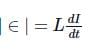where L is the self inductance and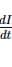is the rate of change of current.

∴ Dimensional formula of  L =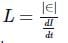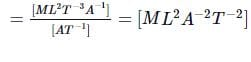31 Year NEET Previous Year Questions: Units & Measurement - Question 5

Of the following quantities, which one has dimension different from the remaining three? 

Detailed Solution for 31 Year NEET Previous Year Questions: Units & Measurement - Question 5

For angular momentum, the dimensional formula is ML2T–1. For other three, it is ML-1T–2.

31 Year NEET Previous Year Questions: Units & Measurement - Question 6

If x = at + bt2, where x is the distance travelled by the body in kilometers while t is the time in seconds, then the units of b is 

Detailed Solution for 31 Year NEET Previous Year Questions: Units & Measurement - Question 6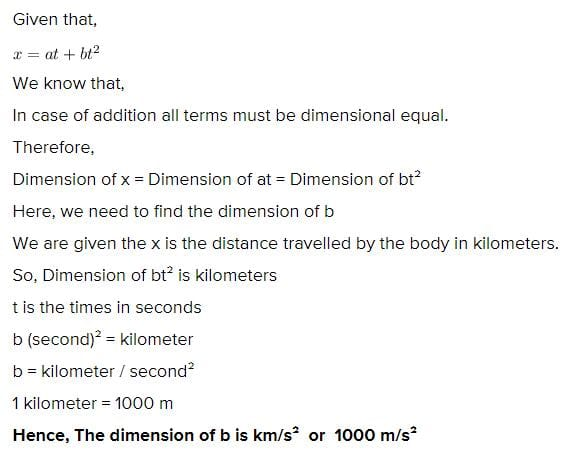31 Year NEET Previous Year Questions: Units & Measurement - Question 7

According to Newton, the viscous force acting between liquid layers of area A and velocity gradient ΔV/ΔZ is given by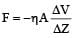where η is constant called coefficient of viscosity. The dimensional formula of η is 

Detailed Solution for 31 Year NEET Previous Year Questions: Units & Measurement - Question 7

Substitute the dimension al formula of F, A, ΔV and ΔZ on both sides and find that for η.

31 Year NEET Previous Year Questions: Units & Measurement - Question 8

The frequency of vibration f of a mass m suspended from a spring of spring constant k is given by a relation of the type f = c mx ky, where c is a dimensionless constant. The values of x and y are

Detailed Solution for 31 Year NEET Previous Year Questions: Units & Measurement - Question 8

f = c mx ky; Spring constant k = force/length. [M0L0T–1] = [Mx (MT–2)y] =[ Mx + y T–2y]

⇒ x+y = 0, -2y = -1 or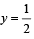Therefore,  x = -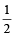31 Year NEET Previous Year Questions: Units & Measurement - Question 9

The dimensional formula of pressure is 

Detailed Solution for 31 Year NEET Previous Year Questions: Units & Measurement - Question 9

[Pressure] = [Force] / [Area]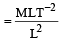= ML–1T–2

31 Year NEET Previous Year Questions: Units & Measurement - Question 10

The dimension al formula for permeability µ is given by 

Detailed Solution for 31 Year NEET Previous Year Questions: Units & Measurement - Question 10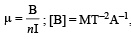[n] = L–1,  [I] = A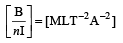31 Year NEET Previous Year Questions: Units & Measurement - Question 11

A certain body weighs 22.42 gm and has a measured volume of 4.7 cc. The possible error in the measurement of mass and volume are 0.01 gm and 0.1 cc. Then maximum error in the den sity will be 

Detailed Solution for 31 Year NEET Previous Year Questions: Units & Measurement - Question 11

D = M/V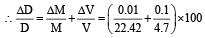=2 %

31 Year NEET Previous Year Questions: Units & Measurement - Question 12

P represents radiation pressure, crepresents speed of light and S represents radiation energy striking unit area per sec. The non zero integers x, y, z such that Px Sy cz is dimensionless are 

Detailed Solution for 31 Year NEET Previous Year Questions: Units & Measurement - Question 12

Try out the given alter natives.
When x = 1, y = –1, z = 1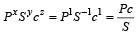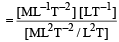= [M0L0T0]

31 Year NEET Previous Year Questions: Units & Measurement - Question 13

The time dependence of a physical quantity p is given by p = p0 exp (– αt2), where α is a constant and t is the time. The constant α 

Detailed Solution for 31 Year NEET Previous Year Questions: Units & Measurement - Question 13

In p = p0 exp (–αt2), αt2 dimension less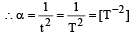31 Year NEET Previous Year Questions: Units & Measurement - Question 14

urpentine oil is flowing through a tube of length l and radius r. The pressure difference between the two ends of the tube is P. The viscosity of oil is given by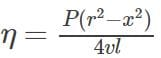where v is the velocity of oil at a distance a from the axis of the tube. The dimensions of η are

Detailed Solution for 31 Year NEET Previous Year Questions: Units & Measurement - Question 14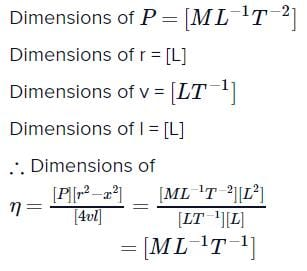31 Year NEET Previous Year Questions: Units & Measurement - Question 15

In a vernier calliper N divisions of vernier scale coincides with (N – 1) divisions of main scale (in which length of one division is 1 mm). The least count of the instrument should be

Detailed Solution for 31 Year NEET Previous Year Questions: Units & Measurement - Question 15

Least count = 1MSD – 1 VSD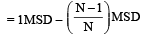(∵ N  VSD = (N – 1)MSD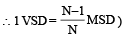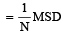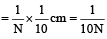31 Year NEET Previous Year Questions: Units & Measurement - Question 16

In a particular system, the unit of length, mass and time are chosen to be 10 cm, 10 g and 0.1 s respectively. The unit of force in this system will be equivalen t to 

Detailed Solution for 31 Year NEET Previous Year Questions: Units & Measurement - Question 16

[F] = MLT–2 = (10g) (10 cm) (0.1s)–2
= (10–2 kg) (10–1m) (10–1s)–2 = 10–1N.

31 Year NEET Previous Year Questions: Units & Measurement - Question 17

Which of the following is a dimensional constant? 

Detailed Solution for 31 Year NEET Previous Year Questions: Units & Measurement - Question 17

A quantity which has dimensions and a constant value is called dimensional constant. Therefore, gravitational constant (G) is a dimensional constant.

31 Year NEET Previous Year Questions: Units & Measurement - Question 18

The percentage errors in the measurement of mass and speed are 2% and 3% respectively.The error in kinetic energy obtained by measuring mass and speed will be 

Detailed Solution for 31 Year NEET Previous Year Questions: Units & Measurement - Question 18

Percentage error in mass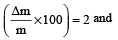percentage error in speed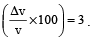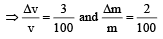Kinetic energy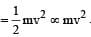∴ Error in measurement of kinetic energy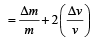By Binomial Function ,

Reqd. error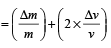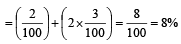∴ %age error = 8%.

31 Year NEET Previous Year Questions: Units & Measurement - Question 19

An equation is given as :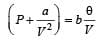where P = Pressure, V = Volume & θ = Absolute temperature. If a and b are constants, then dimensions of a will be 

Detailed Solution for 31 Year NEET Previous Year Questions: Units & Measurement - Question 19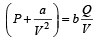According to the principle of homogeinity quantity with same dimension can be added or subtracted.
Hence, Dimension of P = Dimension of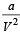⇒ Dimension of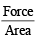= Dimension of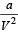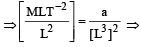a = [M L5 T–2]

31 Year NEET Previous Year Questions: Units & Measurement - Question 20

The density of a cube is measured by measuring its mass and length of its sides. If the maximum error in the measurement of mass and length are 4% and 3% respectively, the maximum error in the measurement of den sity will be 

Detailed Solution for 31 Year NEET Previous Year Questions: Units & Measurement - Question 20

Density =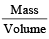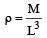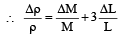% error in density = % error in Mass + 3 (% error in length]
= 4 + 3(3) = 13%

31 Year NEET Previous Year Questions: Units & Measurement - Question 21

Which of the following will have the dimensions of time           

Detailed Solution for 31 Year NEET Previous Year Questions: Units & Measurement - Question 21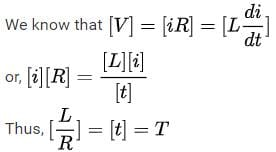31 Year NEET Previous Year Questions: Units & Measurement - Question 22

The force F on a sphere of radius  a moving in a medium with velocity v is  given by F = 6πηav.The dimensions of η are 

Detailed Solution for 31 Year NEET Previous Year Questions: Units & Measurement - Question 22

F= 6πη av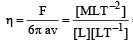= ML–1T–1

31 Year NEET Previous Year Questions: Units & Measurement - Question 23

The dimensional formula for magnetic flux is 

Detailed Solution for 31 Year NEET Previous Year Questions: Units & Measurement - Question 23

Dimension of magnetic flux = Dimension of voltage × Dimension of time = [ML2T–3A–1] [T]  = [ML2T–2A–1]

∵ Voltage =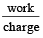31 Year NEET Previous Year Questions: Units & Measurement - Question 24

Which one of the following groups have quantities that do not have the same dimensions? 

Detailed Solution for 31 Year NEET Previous Year Questions: Units & Measurement - Question 24

Force has dimension [MLT–2] while impulse has dimension [MLT–1], both have different dimensions.

31 Year NEET Previous Year Questions: Units & Measurement - Question 25

The dimensions of Planck’s constant are same as    

Detailed Solution for 31 Year NEET Previous Year Questions: Units & Measurement - Question 25

We know that E = hv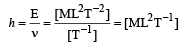Angular momentum = Iω
= [ML2][T–1] = [ML2T–1]

31 Year NEET Previous Year Questions: Units & Measurement - Question 26

The unit of the Stefan-Boltzmann's constant is   

Detailed Solution for 31 Year NEET Previous Year Questions: Units & Measurement - Question 26

E = σAT4
E is energy dissipated per second.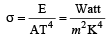31 Year NEET Previous Year Questions: Units & Measurement - Question 27

The unit of permittivity of free space, εo is  

Detailed Solution for 31 Year NEET Previous Year Questions: Units & Measurement - Question 27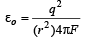⇒ unit of εo is (coulomb)2/ newton -metre2

31 Year NEET Previous Year Questions: Units & Measurement - Question 28

The dimensions of universal gravitational constant are [1992, 2004]

Detailed Solution for 31 Year NEET Previous Year Questions: Units & Measurement - Question 28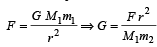∴  dimension of G is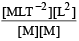= M–1L3T–2

31 Year NEET Previous Year Questions: Units & Measurement - Question 29

The ratio of the dimension of Planck’s constant and that of the moment of inertia has the dimension of 

Detailed Solution for 31 Year NEET Previous Year Questions: Units & Measurement - Question 29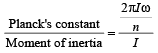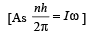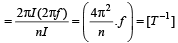31 Year NEET Previous Year Questions: Units & Measurement - Question 30

The velocity v of a particle at time t is given by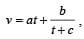where a, b and c are constant.The dimensions of a, b and c are respectively 

Detailed Solution for 31 Year NEET Previous Year Questions: Units & Measurement - Question 30

Dimension of  a. t = dimension of velocity a . t = LT-1 ⇒ a = LT-2
Dimension of c = dimension of t (two physical quantity of same dimension can only be added)

So, dimension of c = T

Dimension of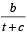= Dimension of v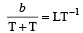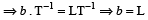So, answer is LT–2, L & T

31 Year NEET Previous Year Questions: Units & Measurement - Question 31

Dimensions of resistance in an electrical circuit, in terms of dimension of mass M, of length L, of time T and of current I, would be 

Detailed Solution for 31 Year NEET Previous Year Questions: Units & Measurement - Question 31

Dimensions of Resistance,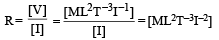31 Year NEET Previous Year Questions: Units & Measurement - Question 32

If the error in the measurement of radius of a sphere is 2%, then the error in the determination of volume of the sphere will be: 

Detailed Solution for 31 Year NEET Previous Year Questions: Units & Measurement - Question 32

Error in the measurement of radius of a sphere = 2%

Volume of the sphere =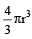∴  Error in the volume =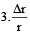= 3 × 2% = 6%

31 Year NEET Previous Year Questions: Units & Measurement - Question 33

Which two of the following five physical parameters have the same dimensions?
(A) Energy density 
(B) Refractive index
(C) Dielectric constant
(D) Young’s modulus
(E) Magnetic field

Detailed Solution for 31 Year NEET Previous Year Questions: Units & Measurement - Question 33

[Energy density] =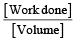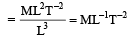[Young’s Modulus] =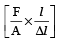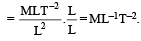31 Year NEET Previous Year Questions: Units & Measurement - Question 34

If the dimensions of a physical quantity are given by Ma Lb Tc, then the physical quantity will be:

31 Year NEET Previous Year Questions: Units & Measurement - Question 35

The dimension ofwhere ε0 is permittivity of free space and E is electric field, is:

Detailed Solution for 31 Year NEET Previous Year Questions: Units & Measurement - Question 35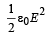represents energy density i.e., energy per unit volume.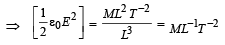31 Year NEET Previous Year Questions: Units & Measurement - Question 36

The dimensions of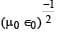are    

Detailed Solution for 31 Year NEET Previous Year Questions: Units & Measurement - Question 36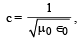, so, dimensions are [LTT–1].

31 Year NEET Previous Year Questions: Units & Measurement - Question 37

The density of material in CGS system of units is 4g/cm3. In a system of units in which unit of length is 10 cm and unit of mass is 100 g, the value of density of material will be [2011M]

Detailed Solution for 31 Year NEET Previous Year Questions: Units & Measurement - Question 37

In CGS system,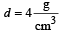The unit of mass is 100g and unit of length is 10 cm, so

density =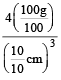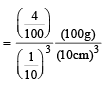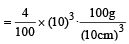= 40 unit

31 Year NEET Previous Year Questions: Units & Measurement - Question 38

The dimensions of (μ0ε0)–1/2 are : [2012M]

Detailed Solution for 31 Year NEET Previous Year Questions: Units & Measurement - Question 38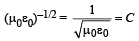: speed of light

where ε0 =  permittivity of free space

μ0 = permeability of free space

So dimension LT–1

31 Year NEET Previous Year Questions: Units & Measurement - Question 39

In an experiment four quantities a, b, c and d are measured with percentage error 1%, 2%, 3% and 4% respectively. Quantity P is calculated as follows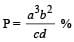error in P is [NEET 2013]

Detailed Solution for 31 Year NEET Previous Year Questions: Units & Measurement - Question 39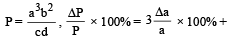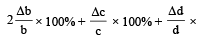100%.
= 3 × 1% + 2 × 2% + 3% + 4% = 14%

31 Year NEET Previous Year Questions: Units & Measurement - Question 40

The pair of quantities having same dimensions is [NEET Kar. 2013]

Detailed Solution for 31 Year NEET Previous Year Questions: Units & Measurement - Question 40

Work = Force ×  displacement Torque = Force × force arm

= mass × acceleration × length

= [M] × [LT–2] × [L] = [M L2T–2]

## Physics Class 11

127 videos|464 docs|210 tests
 Use Code STAYHOME200 and get INR 200 additional OFF Use Coupon Code
Information about 31 Year NEET Previous Year Questions: Units & Measurement Page
In this test you can find the Exam questions for 31 Year NEET Previous Year Questions: Units & Measurement solved & explained in the simplest way possible. Besides giving Questions and answers for 31 Year NEET Previous Year Questions: Units & Measurement, EduRev gives you an ample number of Online tests for practice

## Physics Class 11

127 videos|464 docs|210 tests

### How to Prepare for NEET

Read our guide to prepare for NEET which is created by Toppers & the best Teachers